# Rational Equations Rational Equations Solving Rational Equations is

• Slides: 12Rational Equations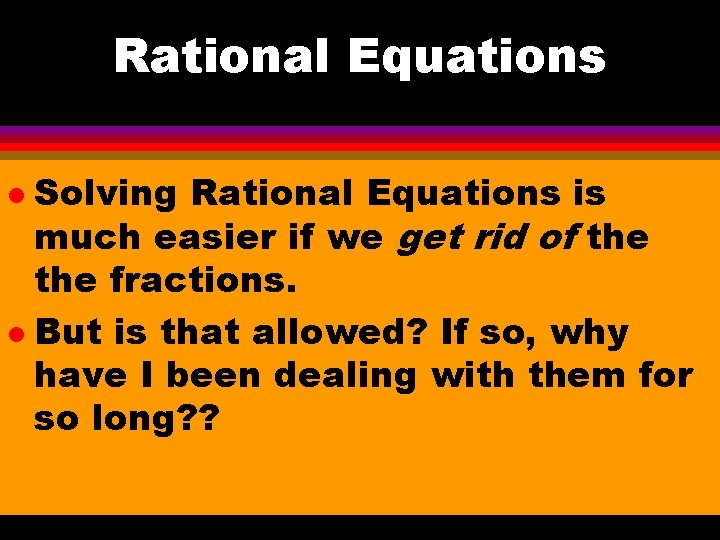Rational Equations Solving Rational Equations is much easier if we get rid of the fractions. l But is that allowed? If so, why have I been dealing with them for so long? ? l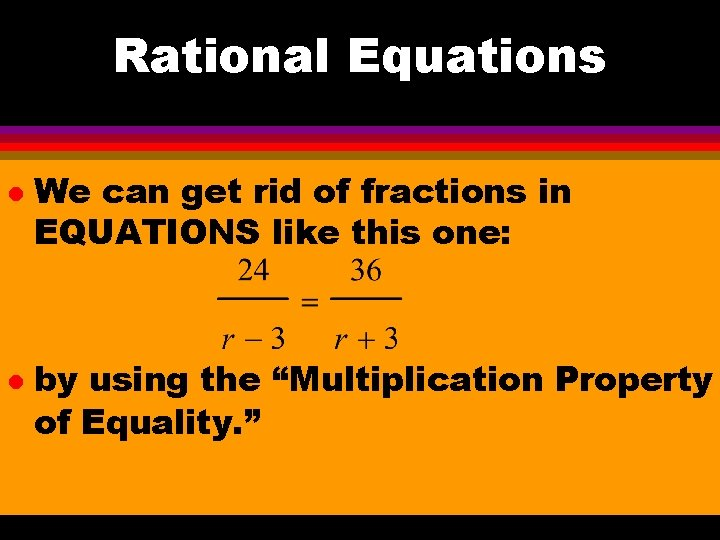Rational Equations l l We can get rid of fractions in EQUATIONS like this one: by using the “Multiplication Property of Equality. ”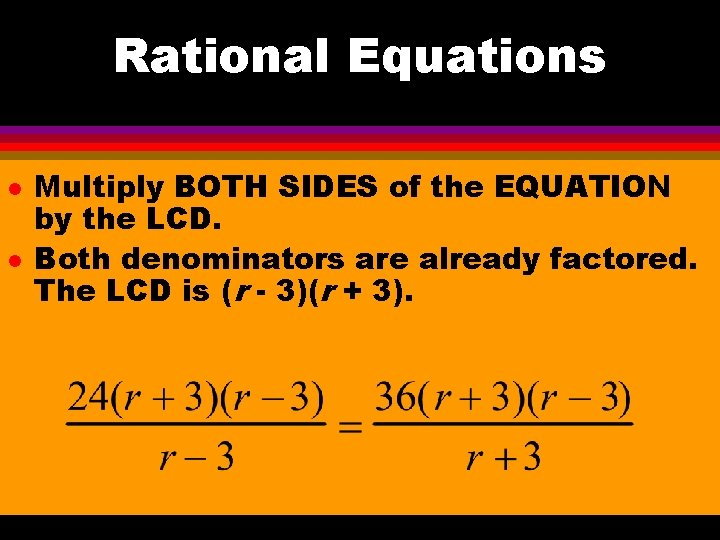Rational Equations l l Multiply BOTH SIDES of the EQUATION by the LCD. Both denominators are already factored. The LCD is (r - 3)(r + 3).Rational Equations l Now the denominators cancel: l Solve the remaining equation: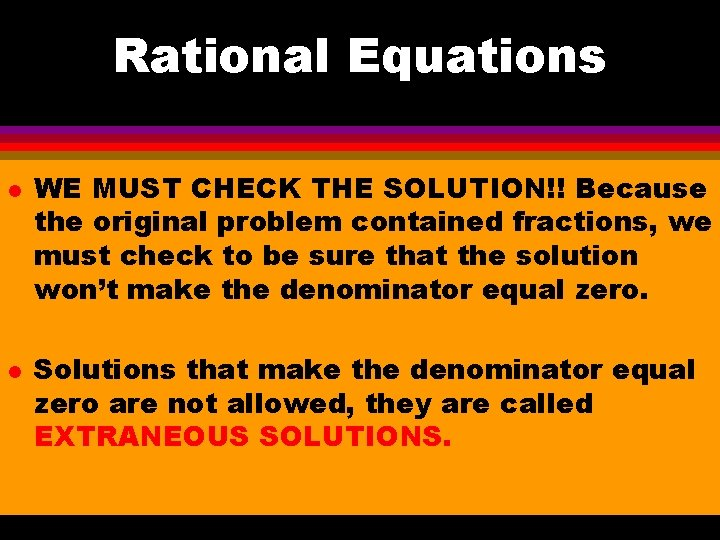Rational Equations l l WE MUST CHECK THE SOLUTION!! Because the original problem contained fractions, we must check to be sure that the solution won’t make the denominator equal zero. Solutions that make the denominator equal zero are not allowed, they are called EXTRANEOUS SOLUTIONS.Rational Equations l Substitute the solution, r =15 into the original equation: This solution checks out fine.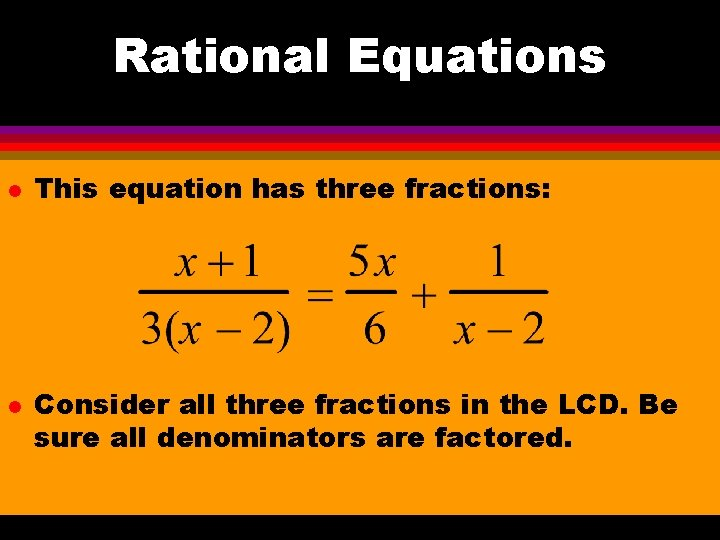Rational Equations l l This equation has three fractions: Consider all three fractions in the LCD. Be sure all denominators are factored.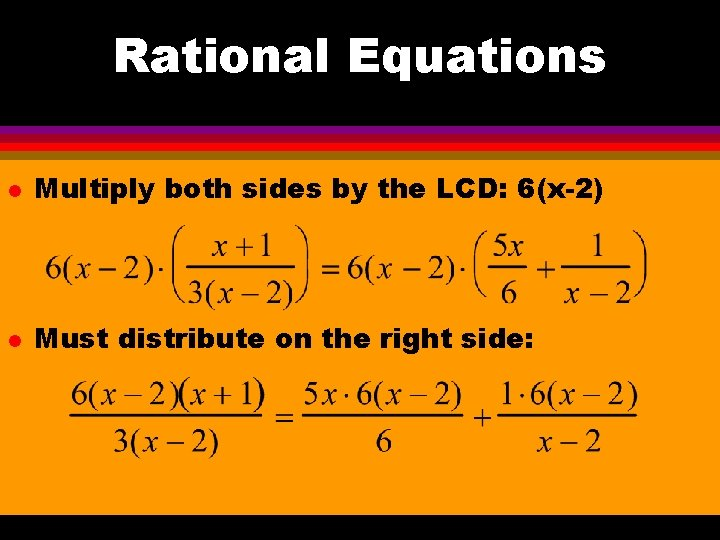Rational Equations l Multiply both sides by the LCD: 6(x-2) l Must distribute on the right side:Rational Equations l Cancel and solve the equation: 2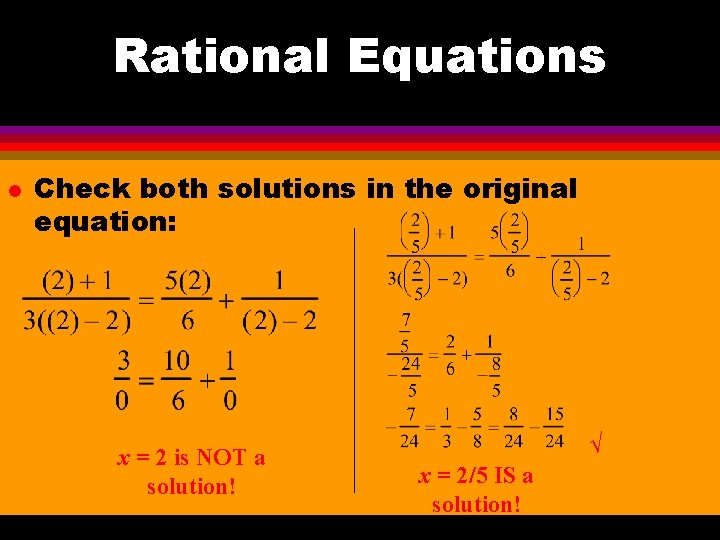Rational Equations l Check both solutions in the original equation: x = 2 is NOT a solution! √ x = 2/5 IS a solution!Rational Equations l l If we can get rid of fractions when they are in equations, why can’t we always get rid of them? The “Multiplication Property of Equality” (multiplying the same term on both sides of an equation) is only true for equations balanced around an = sign.